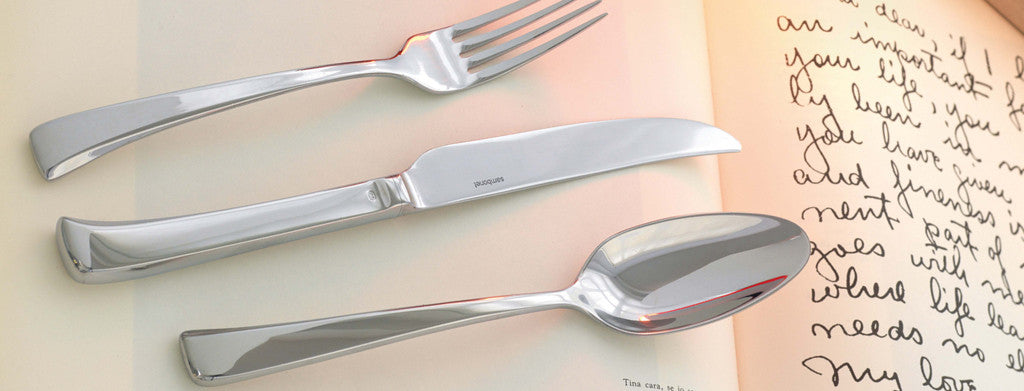Shopping Cart

Refine

View all

PriceCutlery£3.04 each (ex VAT)£3.53 each (ex VAT)£2.25 each (ex VAT)£3.55 each (ex VAT)£2.94 each (ex VAT)£4.89 each (ex VAT)£4.99 each (ex VAT)£3.04 each (ex VAT)£2.25 each (ex VAT)£4.99 each (ex VAT)£3.05 each (ex VAT)£33.24 each (ex VAT)£4.89 each (ex VAT)£3.53 each (ex VAT)£3.53 each (ex VAT)£7.77 each (ex VAT)£5.49 each (ex VAT)£3.09 each (ex VAT)£3.53 each (ex VAT)£7.92 each (ex VAT)£5.49 each (ex VAT)£6.76 each (ex VAT)£4.89 each (ex VAT)£5.59 each (ex VAT)£3.47 each (ex VAT)£4.37 each (ex VAT)£3.84 each (ex VAT)£4.37 each (ex VAT)£3.05 each (ex VAT)£6.76 each (ex VAT)£4.45 each (ex VAT)£3.53 each (ex VAT)£5.49 each (ex VAT)£6.04 each (ex VAT)£4.89 each (ex VAT)£3.09 each (ex VAT)£3.55 each (ex VAT)£2.63 each (ex VAT)£32.86 each (ex VAT)£7.77 each (ex VAT)£4.53 each (ex VAT)£5.90 each (ex VAT)£5.18 each (ex VAT)£7.77 each (ex VAT)£6.91 each (ex VAT)£6.91 each (ex VAT)£6.91 each (ex VAT)£5.75 each (ex VAT)